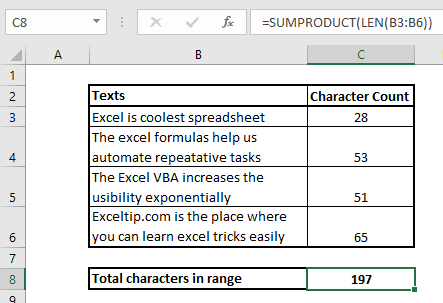# How to Count Total Characters in a Range

We know that the total characters in a cell can be counted using the Excel LEN function. Now to count total characters in range (more than one cell), we just need count characters in each cell then sum them up. Simple. Isn't it?

There are two methods to of doing this. Let's take a look at the first method.

Generic formula for counting characters in a range

 range))

range: It is the range that contains the text.

Example: Count characters in a rangeIn the image above, we have some texts in range B3:B6. We need to count total characters in the range using one formula. We have used the LEN function to show how many characters each cell contains. We can sum them up but we want to use one formula that does not depend on another formula. We use the generic formula mentioned above.

Write this formula in cell C8.

 =SUMPRODUCT(LEN(B3:B6))

Hit enter. We have the total count of characters in the range.How does it work?

The SUMPRODUCT function is an array function that sums up the given array. Where the LEN function returns the length of the string in a cell or given text.

Here we have fed the LEN function with a range of B3:B6. The LEN function returns the length of each cell in an array.

 {28;53;51;65}

Now, this array is fed to the SUMPRODUCT function. The SUMPRODUCT function returns the sum of these values, which is 197.

Alternative Formula:

The alternative way is use of SUM function with LEN function as array formula. It means that we need to use CTRL+SHIFT+ENTER key combination after writing the function.

 {=SUM(LEN(B3:B6))}

So yeah guys, this is how you can get total counts of text in a range. If you have any doubts or any other special query, write it in the comments section below.

Related Articles:

Count total matches in two ranges in Excel | Learn how to count total matches in two ranges using SUMPROUDCT function.

SUMIFS using AND-OR logic | The SUMIFS can be used with OR logic too. The defualt logic SUMIFS uses is AND logic.

SUMPRODUCT with IF logic | Learn how to use SUMPRODUCT with IF logic without using IF function in the formula.

Popular Article:

50 Excel Shortcuts to Increase Your Productivity | Get faster at your task. These 50 shortcuts will make you work even faster on Excel.

The VLOOKUP Function in Excel | This is one of the most used and popular functions of excel that is used to lookup value from different ranges and sheets.

COUNTIF in Excel 2016 | Count values with conditions using this amazing function. You don't need filter your data to count specific value. Countif function is essential to prepare your dashboard.

How to Use SUMIF Function in Excel | This is another dashboard essential function. This helps you sum up values on specific conditions.

Terms and Conditions of use

The applications/code on this site are distributed as is and without warranties or liability. In no event shall the owner of the copyrights, or the authors of the applications/code be liable for any loss of profit, any problems or any damage resulting from the use or evaluation of the applications/code.